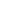# Free Class 10 Difference between two points and section formula Worksheets

Download free printable Class 10 Difference between two points and section formula Worksheets to practice. With thousands of questions available, you can generate as many Class 10 Difference between two points and section formula Worksheets as you want.

## Sample Class 10 Difference between two points and section formula Worksheet Questions

1.

Find the ratio in which the line segment joining the point A(3,-3) and B(-2,7) is divided by x axis. Also find the coordinates of the point of division.

2.

The coordinates of the vertices of triangle PQR are P(7,2), Q(9,10) and R(1,4). If E & F are the mid point of PQ and PR, prove that EF= 1/2 QR.

3.

If the distance of P(x,4) from A(6,2) and B(-2,6) are equal prove that y=2x.

4.

If the coordinate of point P on the line segment joining A(1,2) and B(6,7) such that AP = 2/5 AB.

5.

If the coordinates of points A and B are (-2,-2) and (2,-4) find the coordinates of P such that AP = 3/7 AB, where P lies on the line segment AB.

6.

Point A lies on the line segment PQ  joining P(6,-6) and Q (-4,-1) in such a way that PA/PQ = 2/5. If point P also lies on the line 3x + K (y+1) = 0. Find the value of k.

7.

Show that the points (a,a) (-a,-a) and are the vertices of an equilateral triangle.

8.

If the point C(-1, 2) divides internally the line segment joining the points A(2,5) and B(x,4) in the ratio 3:4. Find the value of

9.

If the vertices of ABC are A(5,-1), B(-3,-2), and C(-1,8), find the length if median through A.

10.

If (3,2) and (-3,2) are two vertices of an equilateral triangle which contains the origin, find the third vertex.

Worksheets by UrbanPro

Our worksheets are designed to help students explore various topics, practice skills and enrich their subject knowledge, to improve their academic performance. Designed by Experts who have extensive experience and expertise in teaching a subject, these worksheets will improve your child's problem-solving skills and subject knowledge in a fun and interactive manner.
Check out our free customized worksheets across school boards, grades, subjects and levels of subject knowledge. You can download, print and share these worksheets with anyone, anywhere, anytime!

Get a custom worksheet to practice!

Select your topic & see the magic.

subjectSelect Chapter(s)

Chapters & Subtopics